## 1. Introduction

One of the main sources of cadastral activities, engineering projects, geographical information system (GIS) applications to be accurate, reliable and sustainable throughout the country is the unique projection coordinates used in scaled maps. In this context, it has been aimed to reduce the distortions occurring in maps to minimum levels by using correction formulas. This is achieved by scale and map harmony. The fact that the coordinate system is not single causes more than one start of coordinates throughout the country. In the zone system, only two neighbouring zones can be converted. If there are more than two zones, it is necessary to switch to another projection system. This leads to an increase in processing load and loss of time due to user errors.

Factors such as geodetic location, size and shape have been taken into consideration in order to minimise distortions in the projection selection of countries. However, due to both military and political aims, projections’ choice for these criteria has been taken into account and the Universal Transverse Mercator (UTM) system is preferred in Turkey. Turkey is a country that extends in the east–west direction. Therefore, the preference of UTM with increased projection distortion in this direction caused the use of more than one UTM zone in the country. Therefore, coordinate unity cannot be established in the ongoing Turkey's National Geographic Information System, the National Spatial and Spatial Data Infrastructure (NSDI) studies and engineering projects exceeding one slice. The aim of this study is to determine medium- and large-scale maps in the country and a projection with a single non-UTM coordinate origin for these projects. Therefore, the most suitable projection system for Turkey is the Lambert conformal conical (LCC2) projection type, in which the distortion values increase in the north–south direction. The studies of the countries on the selection of different projections other than UTM are available in the literature (Bugayevskiy and Snyder, 1995; Cory et al., 2001; Dennis, 2018; Habib, 2008; Hartzell et al., 2002; Huryeu and Padshyvalau, 2007; Vaníček and Najafi-Alamdari, 2004; Veverka, 2004). In this article, it has been aimed to determine a projection system by examining the applicability of LCC2 and external projection systems for Turkey, which lies in the east–west direction, parallel to latitude and located in the mid–latitude belt between the equator and the pole. Thus, a single-beginning and single-zone projection system throughout the country, along both the north–south and east–west directions, and a single coordinate system in all medium- and large-scale sheets are defined.

## 2. Materials and Methods

### Usage and disadvantages of UTM in Turkey

In Turkey, base maps in the scale of 1:25,000 are produced in Gauss–Kruger (GK) mapping system with 6° zones. On the other hand, large-scale cadastral maps in the scale of 1:1000 and standard topo-graphic maps in the scale of 1:5000 and 1:10,000 are produced in GK mapping system with 3° zones (LSMMIPR, 2020).

For Turkey, while the central meridians of GK projection with 3°-wide zones (MUTM) are 27°, 30°, 33°, 36°, 39°, 42° and 45°, these central meridians are 27°, 33°, 39° and 45° for GK projection with 6°-wide zones (UTM). There are four zones for the UTM system and the numbers of these zones are 35, 36, 37 and 38. In order to avoid misunderstandings, zone numbers are added before the easting value in UTM coordinates (Figure 1).

In engineering projects that do not fit into a single zone, if there are overlapping parts with the neighbour zones in their 0.5° or 1° overlap area, the coordinate system is obtained by doing a zone transformation. If the application area overflows the overlap area, zone transformation would not be a suitable process. In this situation, it is not proper to work with a single coordinate system. A transformation from the UTM to different coordinate systems has to be done. The transformation and switching to other coordinate systems cause errors and loss of time because the users do not possess sufficient information. Therefore, the UTM is not sufficient for engineering projects that do not fit into a single zone. Hence, different representation methods should be used for these projects. However, the methods that implement different approaches other than the classical zone width approach have taken their place in the literature (Yildirim, 2004).

### Projection methods and data set for determination of distortions

Given Turkey's geodetic location and considering the origin of a single coordinate system, projection zone width is increased (l ± 3.5°) UTM, Composite (CP), LCC2 and Double (DP). 585 test points have been selected in GRS80 ellipsoid and ITRF96 datum reference surface by creating a geographical grid network (0.5° × 0.5°) within the geographical borders of Turkey (B = [35.5°N–42.5°N]; L = [26°E–45°E]). In order to distribute the distortions that occur in all projections evenly, the starting coordinate has been taken as the centre of the geodetic boundaries (B0 = 39°N; L0 = 35.5°E). Thus, it has been aimed to examine the distortions that may occur in the border regions as they move away from the beginning. Common formulas have been used for the arc to chord (Tt) and distance (Ss) distortions in each projection. For these distortions, each test point has been chosen as a starting point and 45° azimuth angle and 1-km edges have been produced from this point. The coordinates of the second point of these edges have been calculated with the help of the direct solution of geodetic (Vincenty, 1975). After the calculation of projection distortions, arc to chord (Tt) map and distance (Ss) map correction values have been calculated with the help of projection coordinates. Nomenclature of variables used in projection algorithms has been given in the list below:

 (B, L) Ellipsoid geographical latitude and longitude; (φ, λ) Sphere geographical latitude and longitude; (B0, L0) Central meridian (latitude) and longitude of central meridian; (x, y) Projected coordinates or plane coordinates; (N, E) Northing, Easting map of scale or sheet coordinates; q Isometric latitude for ellipsoid: atanh(sin B) – e atanh(e sin B); ω Isometric latitude for sphere: atanh(sin φ); q, l Isometric coordinates; l L – L0; A Ellipsoid azimuth; S Ellipsoid geodetic or distance on ellipsoid; s Plane or projection distance; t Plane or projection azimuth; γ Grid convergence of ellipsoid; (T – t) The arc to chord distortion of projection (A–t–γ); (S – s) Distance distortion of projection (S–s); (T – t)map The arc to chord correction calculated from (x, y); (S – s)map Distance correction calculated from (x, y); a Semi-major axis of ellipsoid; b Semi-minor axis of ellipsoid; c Polar radius of curvature; e2 Eccentricity of ellipsoid squared; e′2 Second eccentricity of ellipsoid squared; e 2.71828182845904523. . . Euler's number; G Meridian arc length from equator to latitude, meridian distance; M Radius of curvature in the meridian: c/(1 + e′2 cos2 B)3/2; N Radius of curvature in the prime vertical: c/(1 + e′2 cos2 B)1/2; R Radius of Gauss sphere: (MN)1/2 = c/(1 + e′2 cos2 B); r Radius of curvature of the parallel (N cos B); η2 e′2 cos2 B; t tan B; m Point grid scale factor; m0 Grid scale factor assigned to central meridian (longitude) or parallels (latitude); (B1, B2) Standard parallels for Lambert conformal conic.

### UTM methods with increased zone width

Inspired by the Mercator projection that had been developed in the 16th century, J. H. Lambert improved the Transversal Mercator (TM) projection for sphere in the 17th century. TM has been developed by C. F. Gauss in the early 19th century, but published by Schreiber and Kruger for ellipsoid. Therefore, TM is also used as GK. Later, ellipsoid formulas were re-examined by Hristow and the univariate and bivariate power series used today were obtained. The current usage type (Easting and Northing) of UTM was developed by the US Army in 1947. Many countries worldwide use the UTM system in mapping applications. Due to the increase in distortion in the east–west direction of the UTM system, 6° zone meridians have been used. In addition, UTM can be modified for large-scale maps and 3° zone meridians can be used regionally (Deakin et al., 2010; Snyder, 1987).

The formulas used in UTM could only be used for the maximum l = ±3.5° from the initial longitude chosen in the 19th century due to the lack of computational techniques. However, with the development of mathematical computer software for numerical computing (MATLAB, Maxima, Mathematica, Maple, etc.), new solutions (Bermejo-Solera and Otero, 2009; Bowring, 1990, 1993a,b; Deakin et al., 2010; Engsager and Poder, 2007; Grafarend, 1995; Guo et al., 2020; Ingwersen, 1996; Karney, 2011; Klotz, 1993; Lee, 1962, 1963; Mittermayer, 1993; Thompson, 1975; Turiño, 2004, 2008) have been developed for l > ±3.5° by increasing the number of coefficients in UTM. The coefficients used in the calculations are presented ready to the user. Mittermayer's method has been preferred in this study as it provides ease of calculation. The correlations in this method are given below:

##### (1)
xmit=G90[q¯+A(q¯,l¯)]
##### (2)
ymit=G90[l¯+B(q¯,l¯)]
##### (3)
A(q¯,l¯)+iB(q¯,l¯)=k=0n¯=9bk(q¯+il¯)k
n¯=n12,n=19
##### (5)
q¯=q¯(q,l)=2πarctan(sinhqcosl)
##### (6)
l¯=l¯(q,l)=2πarctanh(sinlcoshq)
where G90 symbolises the meridian arc length between 0° and 90° latitudes. Meridian convergence and differential scale factor used in determining distortions are calculated with the help of the following equations:
##### (7)
γ=γ(q,l)=arctan(1+C)tanltanhqDDtanltanhq+(1+C)
##### (8)
m=m(q,l)=EG90NcosB(1+C)2+D2
##### (9)
E=2πcoslcoshqcosh2qsin2l
##### (10)
C(q¯,l¯)+iD(q¯,l¯)=k=0n¯=9(2bk+1)(q¯+il¯)2k

The bk coefficients in the Equation (3) and (10), are given in Table 1, in the range of 0–9. The geodetic boundaries of convergence of distortion and meridional in Turkey obtained using this method are shown in Figure 2.

##### Table 1

bk coefficients

kHayford (ED50 Datum)GRS80 (ITRF Datum)
00.0016856285060681990.001678514257669278
1−0.002787990035246185−0.002776158956325202
20.0014061441289513850.001400048837162363
3−0.000359446088023138−0.000357765818293036
40.0000658181553801350.000065443766370321
5−0.000012362414922592−0.000012270448245007
60.0000027050984359610.000002680736087258
7−0.000000594185917121−0.000000588141286135
80.0000001083191740540.000000107115446740
9−0.000000011483903104−0.000000011348588957
##### Figure 2

Distortion sizes for Mittermayer method: (a) scale factor, counter interval 0.0005; (b) meridian convergence, contour intervals 1°; (c) the arc to chord (Tt) distortion, contour intervals 0.2°; (d) the distance (Ss) distortion, contour intervals 75 cm.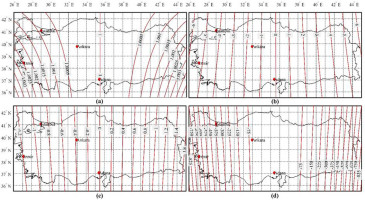### Lambert conformal conic projection

J. H. Lambert developed a single and double standard parallel conical projection for both sphere and ellipsoid in the 17th century. Double standard parallel (B1; B2) LCC2 projection is preferred to work with a single coordinate system in the entire country in order to reduce the distortions that occur from the selected B0 towards north and south in the x-axis direction. Projection coordinate data are calculated with the help of the following equations:

xlcc2=R0¯R¯cosl
ylcc2=R¯sinl
l=lδ
l=δ(LL0)
R¯=R0¯e_[δ(qq0)]
##### (16)
R0¯=N1cosB1δe_[δ(q1q0)]=N2cosB2δe_[δ(q2q0)]
##### (17)
δ=ln(N1cosB1)ln(N2cosB2)q2q1

The meridian convergence and differential scale factor used in determining the distortions are calculated with the help of the following equations (Bugayevskiy and Snyder, 1995; Grossmann, 1976; Hooijberg, 2012; Thomas, 1952; Yang et al., 1999):

γ=l=lδ
##### (19)
m=N1cosB1NcosBe_[δ(qq1)]=N2cosB2NcosBe_[δ(qq2)]

The selection of standard parallels varies according to the shape and size of the study area to be projected. Therefore, the developed equations are as follows:

##### (20)
B1=Bs+BnBsk,B2=Bn+BnBsk
where Bs and Bn symbolise the southern and northern latitude boundaries of the study area respectively. Also, k is a coefficient that changes according to the shape of the region. If the region is rectangular, k = 5; if it is a circle, k = 4; and if it is close to a rhombus, k = 3 (Bugayevskiy and Snyder, 1995). Standard parallels B1 = 37°30′N; B2 = 40°30′N and k = 5 are taken for Turkey. Calculated distortions and meridian convergence are shown in Figure 3.

##### Figure 3

Distortion sizes for LCC2: (a) scale factor, counter interval 0.0005; (b) meridian convergence, contour intervals 1°; (c) the arc to chord (Tt) distortion, contour intervals 0.2″; (d) the distance (Ss) distortion, contour intervals 40 cm.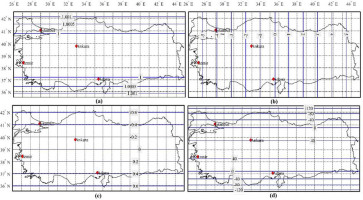### Composite projection (CP)

The CP transcribes the map's geometry to scale, to the map's height-to-width ratio and to the central latitude of the shown area by replacing projections and adjusting their parameters. The CP indicates the entire globe including poles; it portrays continents or larger countries with less distortion and it can morph to the web Mercator projection for maps displaying small areas. By mixing different projections in order to reduce the deformation in scaled maps, applications have been developed for small-scale map applications (Jenny, 2012; Jenny and Šavrič, 2018; Šavrič and Jenny, 2014) as well as large- and medium-scale maps.

CPs (Gojamanov and Ismayilov, 2019; Huryeu and Padshyvalau, 2007, 2008; Padshyvalau et al., 2005; Pędzich, 2005) including Lagrange polyconic projection (Bugayevskiy and Snyder, 1995; Yang et al., 1999) and Chebyshev–Grave criteria (Milnor, 1969; Nestorov, 1997) are defined. CP is a type of projection that minimises distortions that increase in all directions as it moves away from the selected coordinate origin and aims to provide users with formula and calculation convenience. Basic formulas for CP are given below:

xcp=k1x1+k2x2
##### (22)
ycp=k1y1+k2y2
where (x1,y1) and (x2,y2) values are the GK and LCC2 projection co-ordinates used in this study, respectively. Considering the physical size of Turkey's geodetic boundaries, it is seen that there are distortions in both north–south and east–west directions. These two projection methods have been chosen to reduce these distortions in both directions. k1 and k2 are mixed projection coefficients and calculated using the following equation:
##### (23)
dk=n[x1y1][y1][x1]n[x12][x12]
##### (24)
k1=|dk21+dk2|ork1=|1+dk2dk2|
##### (25)
k2=1k1

The value of n in the Equation (23) symbolises the number of points (585 points) calculated in practice. Coordinates of the second projection can also be used in the calculation of dk. In this study, the dk coefficients have been tested in both projections and LCC2 has been preferred because it reduces distortions. In order to prefer CP, the projection equations (x1,y1) and (x2,y2) in Equation (6) should be calculated. For this, isometric coordinate parameter pairs can be written in terms of complex forms in conform projections (Gojamanov and Ismayilov, 2019; Huryeu and Padshyvalau, 2007, 2008; Padshyvalau et al., 2005; Pędzich, 2005):

##### (26)
xj+iyj=F(q+il),j=1,2
##### (27)
Δxj+iyj=k=110ak(Δq+il)k
Δxj=xjx0,x0=G0
##### (29)
Δq=qq0,l=LL0

The following equations are used to avoid calculation overhead in complex functions:

##### (30)
xj=x0+k=110akPk;yj=k=110akQk,j=1,2
##### (31)
Pj=Pj1P1Qj1Q1,P1=qq0,P0=1
##### (32)
Qj=Pj1Q1Qj1P1,Q1=LL0,Q0=0

Since the GK mapping accepts the equator as the beginning of the x-axis, the G0 value must be calculated. On the other hand, in the LCC2 description, since the x-axis is accepted as the beginning of the B0 latitude, it is not necessary to calculate the G0 value (G0 = 0). The ak coefficients are obtained by using the Taylor series at latitude B0 selected by taking l = 0 in accordance with the conformal description conditions of the analytical function derivative (Grossmann, 1976):

##### (33)
a1=dxdq=(dGdq)0=dF(q)dq=F(q)=NcosB}GK
##### (34)
a1=dxdq=(dGdq)0=dF(q)dq=F(q)=R0¯e_δ(qq0)}LCC2
or
##### (35)
an=1n!=(dnxdqn)=(1)n+1n!δnR0¯e_δ(qq0),q=q0}LCC2
##### (36)
a2=12(d2Gdq2)0=12(ddBdGdqdBdq)0,(dBdq)0=N0cosB0M0
##### (37)
a3=16(d3Gdq3)0=16(ddBd2Gdq2dBdq)0

The values of δ and R0¯ in the equations given above for LCC2 are calculated using the Equations (1117). The effect of the ak coefficients on the (x, y) coordinates falls below 1 mm after the 10th iteration, considering the geographical borders of Turkey. These coefficients are difficult to derive and calculate manually. MATLAB software has been used for this process. These coefficients have been calculated using a number of functions (syms, diff and complex) available in the MATLAB library. The ak coefficients for CP are calculated by using Equations (2629) or Equations (3032) instead of Equations (21) and (22) (Gojamanov and Ismayilov, 2019; Huryeu and Padshyvalau, 2007, 2008; Padshyvalau et al., 2005; Pędzich, 2005):

##### (38)
ajCP=k1ajGK+k2ajLCC2

Thus, the ease of calculation for CP is presented to the users with the coefficients in Table 2.

##### Table 2

ak coefficients in metres

GKLCC2CP

kHayford (ED50 Datum)GRS80 (ITRF Datum)Hayford (ED50 Datum)GRS80 (ITRF Datum)Hayford (ED50 Datum)GRS80 (ITRF Datum)
14963550.541404963327.338634961856.517024961633.361224961858.211054961635.05520
2−1561831.78385−1561761.55083−1561480.06295−1561409.82430−1561480.41467−1561410.17603
3−174039.01315−174022.53500327595.12206327580.38357327093.48792327078.78065
4344383.18219344355.40058−51546.55823−51544.23875−51150.62849−51148.33911
5−97650.60029−97639.868016488.613506488.321486384.474296384.19329
6−37371.53049−37368.38135−680.64850−680.61786−717.33938−717.30563
739232.3513439,226.4943261.1993961.19663100.37054100.36193
8−7671.34989−7668.97005−4.81481−4.81460−12.48135−12.47875
9−6850.12639−6849.309000.336710.33670−6.51375−6.51295
105154.825675153.41787−0.02119−0.021195.133655.13225

The meridian convergence and differential scale factor used in determination of the distortions are calculated as follows.

##### (39)
m=K12+K22NcosB,γ=arctanK1K2
##### (40)
K1=j=110jajCPQ(j1),K2=j=110jajCPP(j1)

The distortions and meridian convergence calculated are shown in Figure 4.

##### Figure 4

Distortion sizes for CP: (a) scale factor, counter interval 0.0005; (b) meridian convergence, contour intervals 1°; (c) the arc to chord (Tt) distortion, contour intervals 0.2″; (d) the distance (Ss) distortion, contour intervals 40 cm.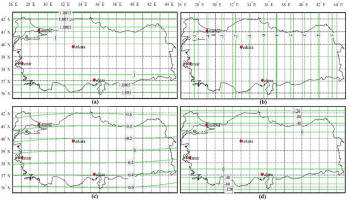### Conical double projection from ellipsoid to sphere and sphere to plane (DP)

The conformal projection of ellipsoid to sphere is fixed and there are many algorithms for conformal projection from sphere to plane. DP consists of two stages: firstly, from the isometric coordinates in the ellipsoid (q, l) to the isometric coordinates in the sphere (ω,Δλ), and then from the geodetic coordinates in the sphere (φ,λ) to the Gaussian sphere (Grossmann, 1976).

##### (41)
ω+iΔλ=K1(q+il)+K2
K1=1+e2cos4B0
##### (43)
K2=arctanh(1K1sinB0)K1q0
ω=K1q+K2
Δλ=λλ0=K1l,λ0=L0
##### (46)
φ=arcsin(tanh(ω))

The double standard parallel projection equations from sphere to plane are as follows:

##### (47)
xdp=R0¯R¯cos(δ(λλ0))
##### (48)
ydp=R0¯R¯sin(δ(λλ0))
R¯=R0¯e_[δ(ωω0)]
##### (50)
R0¯=R1cosφ1δe_[δ(ω1ω0)]=R2cosφ2δe_[δ(ω2ω0)]
##### (51)
δ=ln(R1cosφ1)ln(R2cosφ2)ω2ω1
##### (52)
R1=c/(1+e2cos2B1);R2=c/(1+e2cos2B2)

The meridian convergence and differential scale factor used in determination of distortions are calculated as follows (Bugayevskiy and Snyder, 1995; Grossmann, 1976):

γ=δ(λλ0)
##### (54)
m=R1cosφ1Rcosφe_[δ(ωω1)]=R2cosφ2Rcosφe_[δ(ωω2)]

The calculated distortions and meridian convergence are shown in Figure 5.

##### Figure 5

Distortion sizes for double projection: (a) scale factor, counter interval 0.0005; (b) meridian convergence, contour intervals 1°; (c) the arc to chord (Tt) distortion, contour intervals 0.2″; (d) the distance (Ss) distortion, contour intervals 40 cm.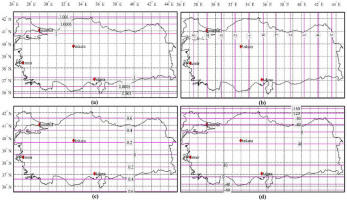### Examination of the projections and a new proposal for Turkey

Considering the distortions in Figures 25, it is seen that the distortion in the Mittermayer method increases as it moves away from the starting meridian in the east–west direction compared to other methods. This means distortion of the size of the map in the whole country in large- and medium-sized maps. When the deformations in the other three methods are examined, it is seen that the edge distortion of CP gives better results between standard parallels compared to LCC2 and DP. In this study, for the first time in Turkey, CPU, GK and LCC2 depictions are used together. The combination of GK and LCC2 equations for the CP depiction equations can be seen as an additional computational burden on users. However, considering the geographical borders of Turkey, the coefficients to be used in the solution of Equations (21, 22) and (2629) are calculated and presented to the users in Tables 2 and 3. Thus, both ease of calculation is provided to the users and the edge distortion between the latitudes (37°30′ −40°30′) falls below 20 cm. The coefficients given in Tables 3 and 4 for CP are valid only for B0 = 39°. For the use of other countries, it is necessary to calculate a new average latitude value of the study area. Calculations for some points for CP are given in Table 5.

##### Table 3

bk coefficients

GKLCC2CP

kHayford (ED50 Datum)GRS80 (ITRF Datum)Hayford (ED50 Datum)GRS80 (ITRF Datum)Hayford (ED50 Datum)GRS80 (ITRF Datum)
12.01468684898033e-072.01477745023166e-072.01537468197565e-072.01546532602613e-072.01537399414265e-072.01546463815034e-07
21.27719412250272e-141.27730899691869e-141.27821479148768e-141.27832976273027e-141.27821377081870e-141.27832874196446e-14
31.90606580617672e-211.90630871057434e-211.08091269101801e-211.08105852690209e-211.08173784413317e-211.08188377708576e-21
42.33217785338216e-282.33259302576196e-281.02832386626856e-281.02850885526054e-281.02962772025567e-281.02981293943104e-28
53.40288110341843e-353.40362666718644e-351.04351317940802e-351.04374783445450e-351.04587254733203e-351.04610771328723e-35
64.92399104670312e-424.92529126527513e-421.10304880523434e-421.10334646159951e-421.10686974747581e-421.10716840640319e-42
77.46687045549524e-497.46916636213681e-491.19929486733841e-491.19967244054757e-491.20556244292657e-491.20594193446916e-49
81.14705437919241e-551.14745779438984e-551.33110423181849e-561.33158317899727e-561.34124367137860e-561.34172617376217e-56
91.79545605447638e-621.79616622523578e-621.50085099606334e-631.50145853535200e-631.51730470561204e-631.51791873906901e-63
102.84208801069137e-692.84333725613141e-691.71339747802719e-701.71416813538263e-701.74010496065608e-701.74088733980856e-70
##### Table 4

Coefficients for correction formulas

ktksk
1−1.230817046856762e-143.410737419053955e-04
2−4.102723489522539e-15−1.230817046856762e-14
3−1.560602822242498e-21−1.230817046856762e-14
4−7.675969852812854e-22−5.117313235208569e-22
57.803014111212491e-221.560602822242498e-21
6−2.882524686831016e-287.799576438254665e-29
79.649191199496467e-292.876383454639922e-28
82.882524686831016e-28−4.824471121337579e-29
##### Table 5

Numerical values for CP (for 1 km) (k1 = 0.001, k2 = 1 − k1)

B [°]L [°]XCP[m]YCP[m]Mm0=1mγ [°](Tt) [″](T-t)mapTt[m](Ss) [m](S-s)mapSs [m]
35.526.0−343547.200−861653.0971.0015020.998500−5.978806280.68060.68050.0000−1.499−1.489−0.010
36.026.5−292879.879−810972.3221.0010180.998983−5.664193310.58500.5852−0.0002−1.015−1.005−0.010
36.527.0−241925.869−760876.1191.0006070.999394−5.349573280.48900.4893−0.0003−0.604−0.595−0.009
37.027.5−190685.986−711365.6361.0002690.999731−5.034946240.39240.3927−0.0003−0.267−0.259−0.008
37.528.0−139161.028−662442.0721.0000050.999995−4.720312230.29520.2955−0.0003−0.0040.003−0.007
38.028.5−87351.773−614106.6790.9998161.000184−4.405671300.19740.1976−0.00020.1850.191−0.006
38.529.0−35258.976−566360.7620.9997021.000298−4.091023500.09890.0990−0.00010.2990.303−0.005
39.029.517116.629−519205.6770.9996621.000338−3.77636888−0.0002−0.00020.00000.3380.341−0.003
39.530.069774.333−472642.8390.9996991.000301−3.46170748−0.1000−0.10020.00020.3010.303−0.002
40.030.5122713.451−426673.7120.9998121.000188−3.14703936−0.2006−0.20090.00030.1870.188−0.001
40.531.0175933.327−381299.8201.0000020.999998−2.83236456−0.3019−0.30240.0005−0.003−0.0040.001
41.031.5229433.329−336522.7411.0002690.999731−2.51768313−0.4040−0.40470.0007−0.271−0.2730.002
42.032.0338751.594−290207.6071.0010400.998961−2.20301786−0.6108−0.61170.0009−1.043−1.0470.004
42.532.5392851.626−246933.1821.0015450.998457−1.88831991−0.7155−0.71660.0011−1.548−1.5530.005
35.533.0−385425.899−227133.6561.0014940.998508−1.573362680.68160.68120.0004−1.490−1.484−0.006
36.033.5−331015.720−180494.9671.0010100.998991−1.258704100.58610.58550.0005−1.007−1.002−0.005
36.534.0−276364.523−134461.1471.0006000.999401−0.944038510.49000.48940.0007−0.597−0.593−0.004
37.034.5−221473.213−89033.2791.0002630.999737−0.629365940.39340.39260.0008−0.261−0.258−0.003
38.035.0−110853.214−43907.7300.9998111.000189−0.314689870.19830.19740.00090.1890.191−0.002
39.035.50.0000.0000.9996591.0003410.000000000.0007−0.00040.00110.3410.3410.000
39.536.055609.95442993.4280.9996961.0003040.31470008−0.0992−0.10040.00120.3030.3030.001
40.036.5111461.66885375.8870.9998101.0001900.62940691−0.1997−0.20100.00130.1890.1880.002
40.537.0167554.386127145.9001.0000001.0000000.94412043−0.3010−0.30240.0014−0.002−0.0040.002
41.037.5223887.372168301.9321.0002680.9997321.25884059−0.4031−0.40470.0015−0.270−0.2730.003
41.538.0280459.918208842.3891.0006150.9993861.57356734−0.5061−0.50770.0016−0.617−0.6210.004
42.038.5337271.342248765.6181.0010400.9989611.88830062−0.6099−0.61160.0018−1.043−1.0470.004
42.539.0394320.989288069.9041.0015450.9984572.20304037−0.7145−0.71640.0019−1.549−1.5540.005
35.539.5−380561.376363342.7201.0014950.9985072.517381640.68270.68120.0015−1.491−1.484−0.007
36.040.5−320609.223451047.1991.0010120.9989893.146763970.58720.58560.0016−1.009−1.002−0.007
36.541.0−262583.287492747.0971.0006030.9993983.461480110.49120.48950.0016−0.600−0.593−0.007
37.041.5−204364.419533824.3641.0002660.9997343.776203400.39460.39290.0017−0.264−0.258−0.006
37.542.0−145953.593574277.9321.0000040.9999964.090933800.29740.29560.0018−0.0030.004−0.006
38.042.5−87351.773614106.6790.9998161.0001844.405671300.19960.19770.00190.1850.191−0.006
38.543.0−28559.910653309.4280.9997031.0002974.720415840.10110.09910.00200.2980.304−0.006
39.043.530421.060691884.9460.9996651.0003355.035167380.0020−0.00020.00230.3350.341−0.006
39.544.089590.217729831.9410.9997031.0002975.34992587−0.0978−0.10030.00250.2970.303−0.006
40.044.5148946.655767149.0650.9998171.0001835.66469127−0.1983−0.20120.00290.1820.189−0.007
42.545.0429557.127780679.3881.0015520.9984515.97971209−0.7135−0.71810.0046−1.555−1.551−0.004

### Calculation of geodetic coordinates from CP inverse projection coordinates

It is necessary to know the geodetic coordinates for transformation from the map coordinates of the newly defined system to the old system and for transition to geocentric coordinates in the Global Navigation Sattellite System (GNSS) application. This is done by inverse transformation from either GK or LCC2 projection coordinates (Equations (4144)):

##### (55)
Δq+il=k=110bk(Δx+iy)k
or
##### (56)
q=q0+k=110bkPk,L=L0+k=110bkQk
##### (57)
Pj=Pj1P1Qj1Q1,P1=Δx,P0=1
##### (58)
Qj=Pj1Q1Qj1P1,Q1=y,Q0=0

For the value of B0 = 39°, the bk coefficients are calculated using the formulas given below:

##### (59)
b1=(dqdG)0=1NcosB}GK
##### (60)
b1=(dqdG)0=1N1cosB1e_δ(qq0)}LCC2
or
##### (61)
bn=1n!=1δnR0¯e_δ(qq0),q=q0}LCC2
##### (62)
b2=12(d2qdG2)0=12(ddBdqdGdBdG)0,(dBdG)0=1M0
##### (63)
b3=16(d3qdG3)0=16(ddBd2qdG2dBdG)0

All calculated bk coefficients are given in Table 3. Calculation of the values of δ and R0¯ used in the above equations are given in Equations (1117). The following equations are used for transition from isometric latitude to geodetic latitude given in Equations (5558) (Kaya, 1994):

##### (64)
B=χ+1/2sin2χ[e2+e4(cos2χ16sin2χ)++e6(cos4χ56sin2χcos2χ+130sin4χ)++e8(cos6χ2sin2χcos4χ+49120sin4χcos4χ+31252sin2χ)]
##### (65)
χ=arcsin(tanhq)

Also, bk coefficients can be obtained by using Equation (66):

##### (66)
bjCP=k1bjGK+k2bjLCC2

The reverse conversion process is performed using Equations (5558) and (66). This process is more practical for users. The coefficients calculated from Equations (5963) and (66) are shown in Table 3.

### Correction formulas of CP

In engineering projects where distance and direction are measured, it is necessary to reduce lengths and direction. Since map coordinates are used in the projects, giving the reduction formulas over these coordinates provides ease of calculation for the users. The reduction formulas commonly used for conformal descriptions (Draheim, 1953) can also be used for CP:

##### (67)
(Tt)map=t1x1Δy+t2ΔxΔy+t3x1y1Δx+t4x12Δy++t5y12Δy+t6x12y1Δx+t7y13Δx+t8x1y12Δy
where:
##### (68)
t1=3t2;t2=1+η026N02;t3=t0(1+η02)2N03;t4=t0(13η024η04)4N03;t5=t32;t6=40+108η02+63η043t0236N04;t7=10η027η04+3t0212N04;t8=t6
##### (69)
(Ss)map=s(s1+s2x12+s3x1Δx+s4x13++s5x1y12+s6x14+s7x12y12+s8y14)
where:
##### (70)
s1=N0cosB0b1CP1;s2=s3=t1;s4=t0(13η024η04)6N03;s5=t3;s6=5+20η02+10η043t0224N04;s7=13η028η04+9t024N04;s8=10η02+10η043t0224N04

The Δx(x2x1) and Δy(y2y1) reductions are the difference between the map coordinate values of the edge to be calculated. In order to provide ease of calculation to the users, tk and sk coefficients in Equations (6668) are calculated in B0 = 39° latitude and ITRF96 datum are given in Table 4. These coefficient values that can be used for up to 2 km in length can be measured in the land of Turkey's geodetic boundaries.

These differences Tt = (Tt) – (Tt)map and Ss = (Ss) – (Ss)map are calculated on the sides of 1 and 2 km and are given in Figure 6. It is observed that the edge distortion (Ss) in Equations (6668) is achieved with an accuracy of 1 cm for 1 km and less than 2 cm for 2 km. In addition, the map distortion (Tt) in Equations (6668) provides an accuracy of less than 0.003″ for 1 km and 0.006″ for 2 km. In this context, Equations (6668) are sufficient for the location accuracy of the angle and distance measurements made in the detail acquisition of projects using large-scale maps and in the application to the terrain.

##### Figure 6

Correction values based on correction formulas for accuracy analysis in CP: (a) Ss difference for 1 km, counter interval 2 mm; (b) Ss difference for 2 km, counter interval 2 mm; (c) Tt difference for 1 km, contour intervals 0.001″; (d) Tt difference for 2 km, contour intervals 0.001″.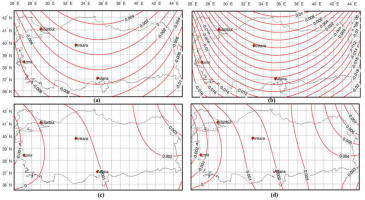### Choice of scale correction factor (m0) and false origin for Turkey

Since large-scale maps (1:1000–1:10,000) cover small areas, m0 is taken as 1 for the whole country (Figure 4a). Thus, in engineering projects that require detailed measurement from the field and application to the field, the land and the map remain the same in 1:1 scale. This provides the users with the ease of completing the application without any additional transformation process in the application of the data produced from large-scale maps to the terrain. However, this is not the case with medium-scale maps (1:25,000–1:250,000). In these maps, due to the area they occupy, distortion increases in the near regions of the depiction centre and as they move away from the depiction centre. For the CP representation, these maps also increase as distortions increase from the chosen initial latitude to the north and south, and particularly at the system edges. It may be possible to keep this growth within a certain boundary by preserving the conditions of representation, and especially to reduce it within the country borders. So, m could be slightly reduced for the whole country. For this, m0 must be equal to 1 at xmax/2. Thus, in the initial latitude, m0 will be slightly smaller than 1, but in the border latitudes (m − 1), the difference will be halved. xmax/2 corresponds to the latitude B = 40.50° and m0 = 1 for CP. The m0value can be chosen as 0.9995, the average value between B = 40.5° and Bmax = 42° in CP (Figure 7).

In order to not work with negative coordinates in scaled maps, at the beginning of the coordinate of the description, negative x values south of latitude B0 = 39° and negative y values west of longitude L0 = 35.5° should be positive. For this, a fixed value (false) must be specified to add to all projection coordinates (xcp, ycp). Negative values of CP coordinates are xmax = −388 km and ymax = −861 km in Turkey (Table 5). Therefore, false northing = 500,000 m and false easting = 1,000,000 m values are determined for positive coordinates. Thus, scale maps for CP (scale map) or sheet (sheet) coordinates can be calculated in Turkey:

##### (71)
Northing(N)=m0xCP+500,000
##### (72)
Easting(E)=m0yCP+1,000,000

In these equations, m0 value is taken as 1 for large-scale maps and 0.9995 for medium- and small-scale maps.

The meanings of the parameters symbolised in Table 5 are explained in Section 2.2. This table has been produced by taking into account the latitude and longitude limit values of Turkey. Distortion values have been calculated in CP as a result of calculations made using MATLAB software.

## 3. Results and Discussion

In this study, projections that can be used outside the UTM system with the beginning of seven meridian zones are examined in Turkey. As it is known in the UTM system, zone transformation is performed in projects in two zones, and transition to a different projection is performed for projects falling into more than two zones. This leads to an increase in working load and working errors caused by users. In this context, Mittermayer with increased meridian zone width, CP, double standard parallel LCC2 and ellipsoid to sphere, spherical to plane conformable DP, which are suitable for the geodetic location and shape of our country, except UTM, have been examined in detail.

In this study, the applicability of CP, which is used for small-scale maps among the discussed projections, for large- and medium-scale maps has been investigated for the first time. Also, for the first time in CP, GK and LCC2 projections have been mixed in order to reduce distortions in all directions. The distortions of the four projection methods have been calculated with the test points determined in the 0.5° × 0.5° geodetic grid covering the geodetic borders of Turkey. According to the results, it has been observed that CP especially has less length distortion compared to LCC2. Thus, it is proven that a projection may also use CP and LCC2, except for Turkey.

Some definitions need to be made in order to be able to use CP in country mapping system, medium- and large-scale maps and engineering projects, which include detail and application processes. These definitions are the transformation of geographic and projection coordinates, direction and length correction formulas, scale correction factor (m0), false easting and false northing, respectively. Also, it is defined as the coefficient in terms of providing convenience to users in this study in Turkey. It is sufficient to calculate these coefficients once according to the initial latitude (B0) and they are valid everywhere within the geodetic boundaries of the country. These coefficients are calculated in ITRF96 and ED50 datum and given to users in this study.

Geodetic–projection coordinate transformation in CP is calculated with the help of Equations (2629, 38). The coefficients are given in Table 2. In addition, the Geodetic–projection coordinate mapping is performed using Equations (5558, 66). Coefficients are given in Table 3. Direction and length corrections are calculated with Equations (6769) and the coefficients are given in Table 4. Equations (6769) are sufficient to determine the position accuracy of the angle and distance measurements made with the total station in places where GNSS cannot be measured in the detail acquisition and application of the projects produced on large-scale maps.

## 4. Conclusions

As is the case in Turkey, slice transformation should be performed for projects in two slices in the UTM system and a different projection system should be used for projects with more than two slices. This situation causes the working load to increase and user-based errors to occur. In this study, projections that can be used outside of UTM are examined in Turkey. Mittermayer, mixed projection (CP), double standard parallel LCC2 and conformal DP to ellipsoidal sphere and from sphere to plane, suitable for Turkey's geographical location and shape, have been studied in detail.

According to the results obtained, map projection CP with the least distortion values in both east–west and north–south directions has been selected. With the CP selection, a single coordinate system has been determined for medium- and large-scale maps. Projection correction formulas, scale factor and false origin have been calculated for map coordinates in CP. These distortions are obtained with a difference of less than 1 cm for 1 km long sides and less than 0.003″ for the azimuth value of this side, when the correction formulas are used. In addition, in this study, equations have been produced in the form of coefficients in order to provide convenience to the users. These coefficients are calculated separately for ITRF and ED50 datums according to the initial latitude (B0) and it is sufficient to calculate once. These coefficients are valid everywhere within the geographical boundaries.